ABAP整型类型的几种位操作 - OR, AND, XOR

+关注继续查看

For training purpose I need to explain to my ABAP team colleagues about how bitwise operation on Integer in Java like below is done.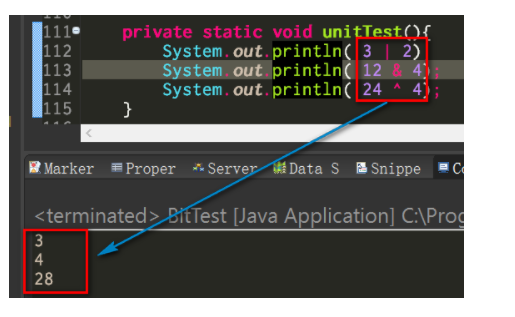And since the bitwise operation in ABAP can only support data type X and XSTRING,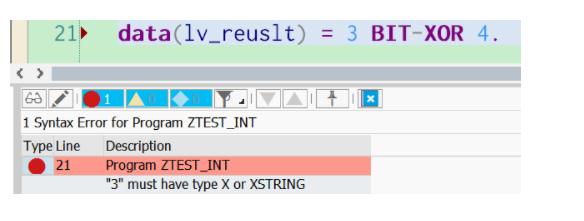so now I create a prototype which can support bitwise operation OR, AND, XOR on int4 in ABAP for teaching purpose.

Still remember the wrapper class Integer in Java which is explained in my blog Integer in ABAP, Java and JavaScript?

I just create a wrapper class ZCL_INTEGER in ABAP by following the design idea used in Java.

Usage of ZCL_INTEGER

Here below the usage of this wrapper class is explained.

Get instance of ZCL_INTEGER

In Java you use the following code to get an instance of wrapper class Integer: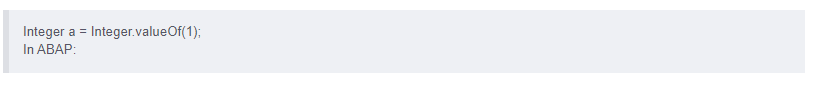data(int1) = zcl_integer=>value_of( 3 ).

data(inta) = zcl_integer=>value_of( 3 ).

data(int2) = zcl_integer=>value_of( 2 ).

ASSERT int1 = inta.The same primitive int value will always lead to exactly the same instance of ZCL_INTEGER. I achieve this by using an internal table MT_CACHE in ZCL_INTEGER as a cache.

Get integer’s binary representation

A string for the integer’s binary format is returned by simply calling method get_binary_format.

zcl_integer=>value_of( 3 ).

data(int2) = zcl_integer=>value_of( 2 ).

WRITE:/ int1->get_binary_format( ).

WRITE:/ int2->get_binary_format( ).

Result: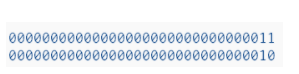Bitwise operation

Use instance method OR, AND and XOR accordingly.

Test code:data(int1) = zcl_integer=>value_of( 3 ).

data(int2) = zcl_integer=>value_of( 2 ).

data(int3) = zcl_integer=>value_of( 12 ).

data(int4) = zcl_integer=>value_of( 4 ).

data(int5) = zcl_integer=>value_of( 24 ).

data(int6) = zcl_integer=>value_of( 4 ).

WRITE:/ int1->or( int2 )->get_raw_value( ).

WRITE:/ int3->and( int4 )->get_raw_value( ).

WRITE:/ int5->xor( int6 )->get_raw_value( ).Same output as Java: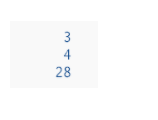Now let’s try to do more interesting task: swap the two integer without using intermediate variableDATA(a) = zcl_integer=>value_of( 20 ).

DATA(b) = zcl_integer=>value_of( 30 ).

a = a->xor( b ).

b = a->xor( b ).

a = a->xor( b ).

WRITE:/ a->get_raw_value( ).

WRITE:/ b->get_raw_value( ).Test output, swap is done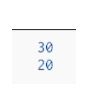Currently this toy only supports unsigned integer.

Please manually create these three simple DDIC objects before you activate the code: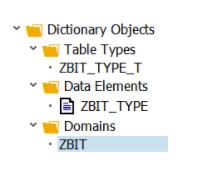ZBIT domain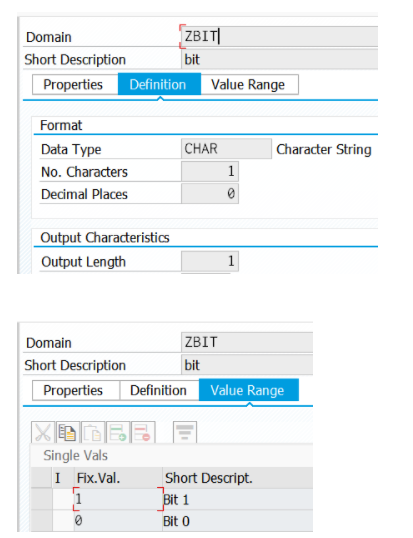Data element for BIT type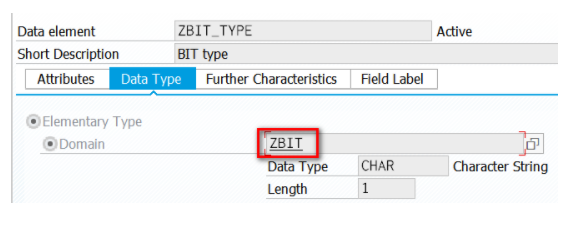And table type: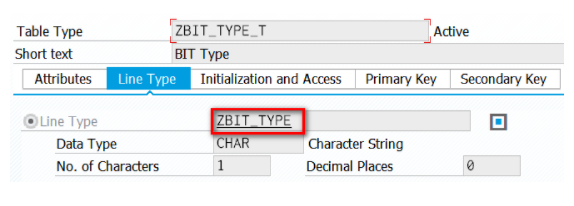Further reading

I have written a series of blogs which compare the language feature among ABAP, JavaScript and Java. You can find a list of them below:

Lazy Loading, Singleton and Bridge design pattern in JavaScript and in ABAP

Functional programming – Simulate Curry in ABAP

Functional Programming – Try Reduce in JavaScript and in ABAP

Simulate Mockito in ABAP

A simulation of Java Spring dependency injection annotation @Inject in ABAP

Singleton bypass – ABAP and Java

Weak reference in ABAP and Java

Fibonacci Sequence in ES5, ES6 and ABAP

Java byte code and ABAP Load

How to write a correct program rejected by compiler: Exception handling in Java and in ABAP

An small example to learn Garbage collection in Java and in ABAP

String Template in ABAP, ES6, Angular and React

Try to access static private attribute via ABAP RTTI and Java Reflection

Local class in ABAP, Java and JavaScript

Integer in ABAP, Java and JavaScript

Covariance in Java and simulation in ABAP

Various Proxy Design Pattern implementation variants in Java and ABAP

Tag(Marker) Interface in ABAP and Java

Bitwise operation ( OR, AND, XOR ) on ABAP Integer

An interview question: Compare two integers without +,-,*,/ or > and <26793 010084 014498 010883 0Xshell使用SSH远程登录阿里云ECS服务器CentOS7
13111 011818 013889 0windows server 2008阿里云ECS服务器安全设置

9161 04659 022403 0
2316

0

+ 订阅

《2021云上架构与运维峰会演讲合集》

《零基础CSS入门教程》

《零基础HTML入门教程》